# Solving Equations Involving Like Terms

 Once you understand the basics of solving equations, you can learn to solve longer and more-involved equations. What do you think would be the first step in an equation like this one? 5x+8x-2=10 If you said that you should first add 5x and 8x, you are right. When you have like terms on the same side of the equation, the first step is to combine them. Let's finish the problem: 5x+8x-2=10 13x-2=10 13x-2+2=10+2 13x=12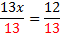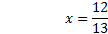We can check our answer if we want, but it starts to get a little more complex. The easiest way to check the answer is to use a calculator. Let's look at one more example: 4y-8+6y=-4 10y-8=-4 7x-5=-8 10y-8+8=-4+8 10y=4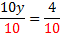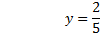Practice:Solve the following equations 1) 8y+2y-6=10 2) 6x+3-2x=7 3) 4a-6a+2=5 4) 3=2x+5x+1 5) 3n+n-2=0 Answers: 1)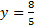2) x=1 3)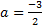4)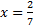5)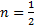Related Links: Math Algebra Factors